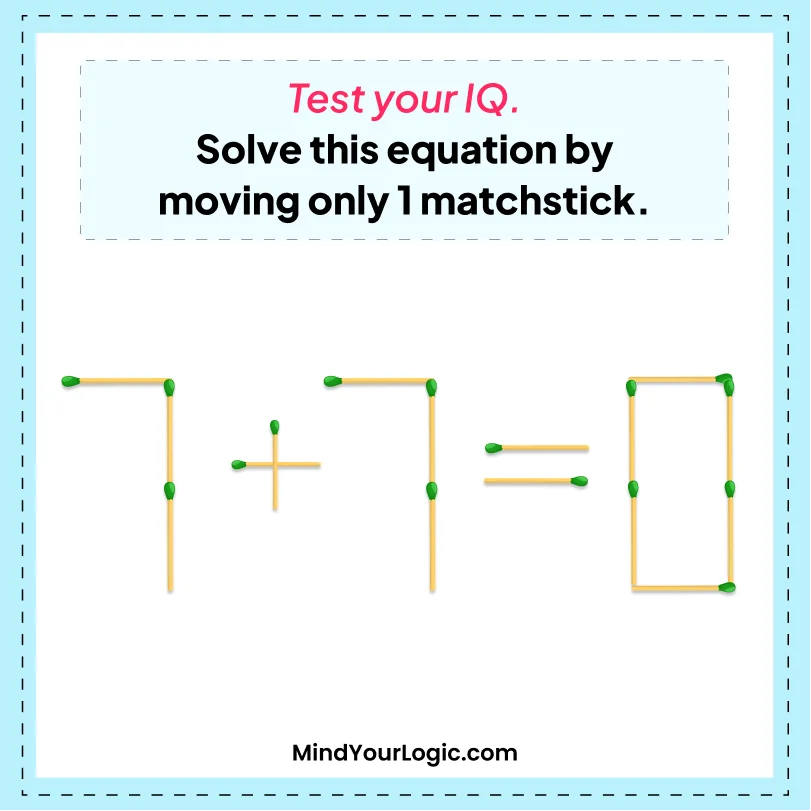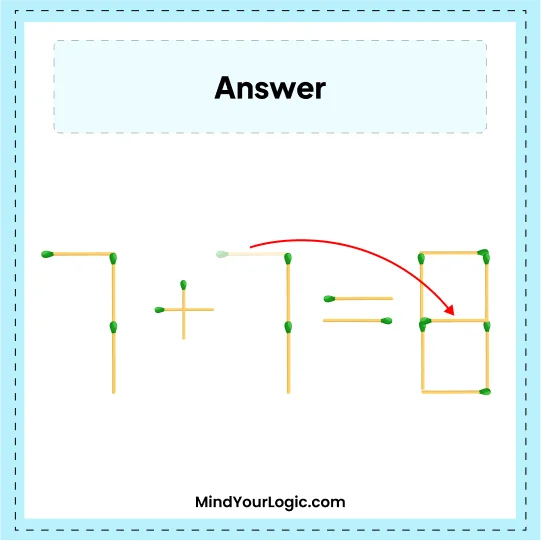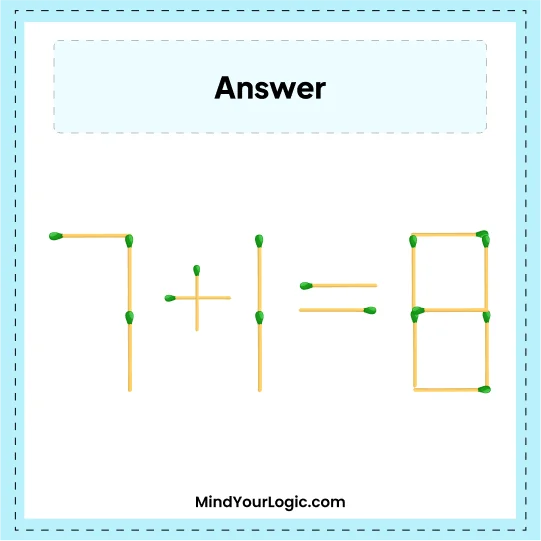# 7+7=0 - Matchstick Puzzle

###### 33.Matchstick Puzzles
`Test your IQ Try to solve this equation by moving  only one matchsticks. `•

Explanation :

` Move one stick from seven and place it in between zero, it will become 8. The correct equation we get is 7 + 1 = 8.`